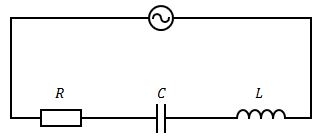# Difference Between Impedance and Resistance

## Main Difference – Impedance vs. Resistance

Impedance and resistance are terms that describe an opposition to a current’s flow through a circuit. The main difference between impedance and resistance is that resistance is a property that depends only on the material that the component is made of, its dimensions and the temperature. For ideal conductors, a change in current through the component does not cause a change in the resistance of that component. Impedance encompasses not only resistance but also reactance: the property that describes how a component opposes any changes in the current, such as the change in current in AC circuits.

## What is Resistance

Resistance$R$ is defined as the ratio of potential difference$V$ across a component to the current$I$through that component:$R=\frac{V}{I}$

Resistance originates when electrons flowing across a conductor collides with lattice ions in the material. Therefore, resistance depends on the resistivity of the material and the dimensions of the component. Resistance also changes with temperature because temperature affects the energy of lattice ions and the electrons. If you connect a resistor to an AC circuit and change the frequency of the AC supply, the resistance of the resistor is not going to change.

## What is Impedance

Capacitors and inductors are components with reactance. This is a property that causes them to oppose changes in current when they are connected in AC circuits. Reactance depends on the frequency of the AC current. For example, for a capacitor with capacitance$C$ in an AC circuit with a frequency$f$, the capacitive reactance$X_C$ is given by$X_C=\frac{1}{2\pi fC}$

For an inductor with inductance$L$, it is given by$X_L=2\pi fL$

Impedance is a quantity that takes into account both resistance and reactance. It is commonly expressed as a complex number because it is composed of a part that does not change (resistance, making up the real part of the number) and also a part that changes periodically (reactance, making up the imaginary part of the number).

The complex impedance ($Z$) is given by:$Z=R+j\left( X_L-X_C\right)$

The magnitude of an impedance is given by$\left| Z\right| =\sqrt{R^2+{\left( X_L-X_C\right)}^2}$.

The phase angle of an impedance$\theta$ (how much the current will lag behind the voltage) is given by$\mathrm{tan}^{-1}\left( \frac{X_L-X_C}{R}\right)$

For example, for the circuit shown belowAn AC circuit containing a resistor, capacitor and an inductor

The magnitude of impedance is$Z=\sqrt{R^2+{\left( 2\pi fL-\frac{1}{2\pi fC}\right)}^2}$.

Phase angle is$\mathrm{tan}^{-1}\left( \frac{2\pi fL-\frac{1}{2\pi fC}}{R}\right)$.

## Difference Between Impedance and Resistance

### Type of Measurement

Resistance does not take into account the opposition to changes in current. Resistance is a property that depends only on the material that the component is made of, its dimensions and the temperature.

Impedance takes into account both resistance as well as reactance (opposition when current changes).

### Type of Circuit

Resistance is a property of both AC and DC circuits.

For a DC circuit, Impedance is simply its resistance.  For an AC circuit, it is a quantity that changes with the frequency of the AC current.